Single Phase Half Wave Rectifier

# Single Phase Half Wave Rectifier - Notes | Study Power Electronics - Electrical Engineering (EE)

 1 Crore+ students have signed up on EduRev. Have you?

What is Rectifier?

• The rectifier is the device that converts alternating current(AC) into direct current(DC). The alternating current periodically reverses its direction whereas the direct current does not periodically change its direction.

What is Half-Wave Rectifier?

• The half wave rectifier passes one half cycle of the alternating current and blocks the other half cycle. Thus in one complete cycle of the AC waveform, the half-cycle is passed. In a half wave rectifier, only one diode is used to convert AC into DC.

Single Phase Half Wave Rectifier Theory

How does Single Phase Half-Wave Rectifier functions?

• The main part of half wave rectifier is the diode. The diode conducts if the anode voltage is more than the cut-in voltage of the diode. The cut-in voltage of silicon and germanium diode is 0.7 and 0.3 Volts respectively. If the anode voltage is more than 0.7 volts, the diode starts conducting. In power electronics, silicon diodes are generally used because they have a low forward resistance which makes them suitable for delivering high forward current.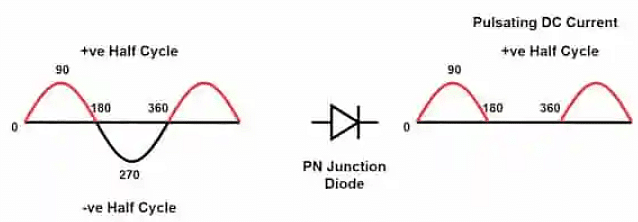• During one positive half cycle, the diode conducts and passes the waveform for the period from o to π. The diode does not conduct for the period from π to 2π because the anode potential is less than the cut-in voltage of the diode. The output waveform of the diode is the DC voltage since it has positive pulses. The output DC waveform has more ripples and it is not smooth.
• We can get negative DC voltage by making the diode conduct during the negative half cycle of the AC waveform.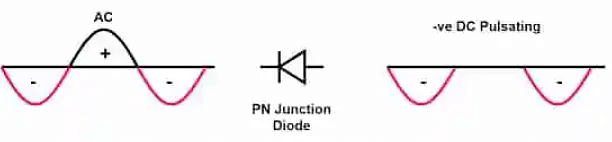• The diode conducts during the negative half cycle of the AC waveform. During the positive half cycle, the diode is in reverse bias and no current flows through the diode.

Circuit Diagram of Half Wave Rectifier

The circuit diagram of Half wave rectifier is as shown below.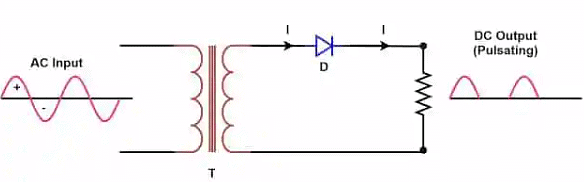The half wave rectifier circuit consists of three main parts.

• Transformer
• Diode

The voltage is applied at the primary side of the transformer. The low voltage is induced in the secondary of the transformer according to the turns ratio of the transformer. The voltage is reduced to get desired DC voltage. The simplified equivalent secondary circuit of the rectifier is as below.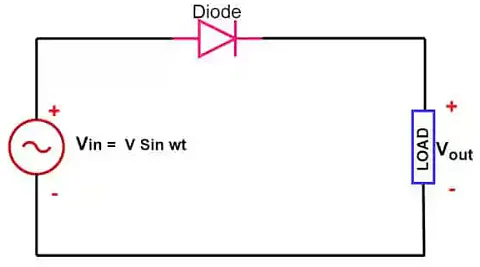• During the positive half cycle of the AC waveform, the voltage at the anode of the diode is more than its cut-in voltage and the diode becomes forward biased and starts conducting. Thus in the positive half cycle diode remains in the conducting state and current flows through the diode. The diode has very low forward resistance during its forward biasing state, and the diode behaves as a short circuit, considering zero forward resistance.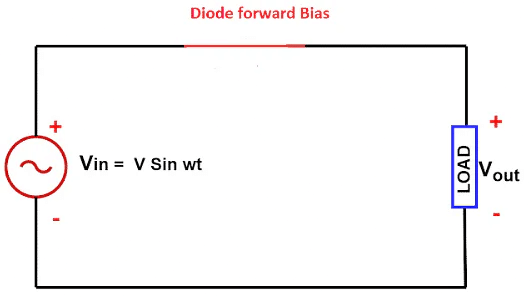• The diode stops conducting when the AC waveform crosses zero and goes towards the negative half cycle. During the negative half cycle, the diode is in reversed bias and no current flows through the diode. During the negative half cycle, the diode is an open circuit. The half wave rectifier equivalent circuit is as given below.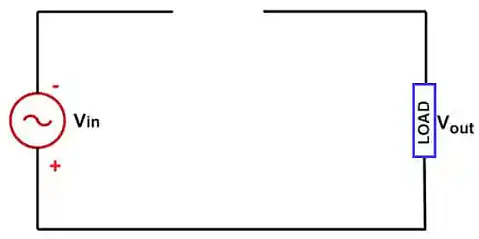• During the negative half cycle, the reverse resistance of the diode is very high and it acts as an open circuit and, the diode does not allow current to pass through it.

Single Phase Half Wave Rectifier Formulas

• We will discuss how the various formulas of half wave rectifier used for assessing the rectifier performance.

Average value or DC value of Single Phase Half Wave Rectifier

• We can calculate the average or effective DC output of half wave rectifier by taking the average of the DC output voltage. The instantaneous value of current at the output of the rectifier is i = ImSinωt. The average load current across the load resistance is equal to: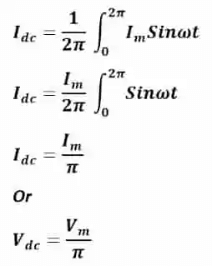RMS Value of Single Phase Half Wave Rectifier

• For a half-wave rectifier, the RMS load current (Irms) is equal to the average current (IDC) multiple by π/2. Hence the RMS value of the load current (Irms) for a half wave rectifier is: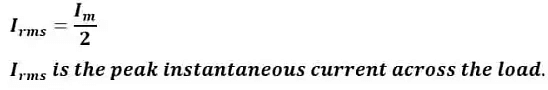Ripple Factor of single Phase Half Wave Rectifier

• The ripple factor shows the effectiveness of rectification. The output of the rectified DC has an AC component as well. The AC component is undesirable in the rectified DC output and the AC component available in the rectified DC is ripple. It is practically impossible to eliminate the AC component in the rectified DC output. However, the lesser ripples in the rectified waveform make the output DC smoother.
• Ripple factor is the ratio of AC component available in the rectifier output to the average value of DC output.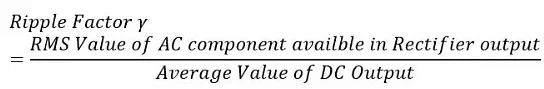• We can express the ripple Factor of the Half Wave Rectifier by the following mathematical expression.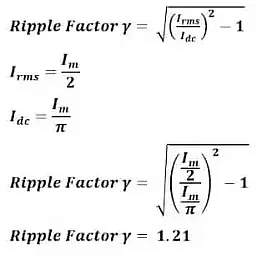• The ripple factor of the half wave rectifier is 1.21. It is possible to lower the ripples by the installation of a capacitor and inductor as a filter circuit.

Form Factor

The ratio of RMS value and the average value is the form factor.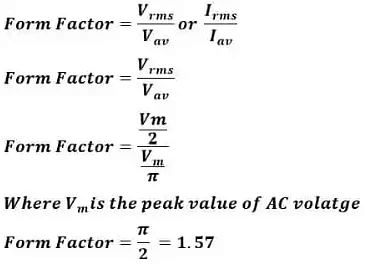Efficiency of Half Wave Rectifier

• The ratio of output DC power to the input AC power is the rectifier efficiency. The formula for the efficiency is equal to:
η = Pdc/Pac
• The efficiency of half wave rectifier is equal to 40.6%.

Peak Inverse Voltage of Half Wave Rectifier

• The peak inverse voltage of the diode is the maximum voltage that a diode can withstand during reverse bias conditions. If the diode gets voltage above peak inverse voltage(PIV), the diode is apt to fail.

Half Wave Diode Rectifier Applications

• Power rectification:  We use Half wave rectifier for rectification of AC to get DC Voltage.
• Signal demodulation:  Through process of demodulation, it is possible to recover the original signal sent from the transmitter end. A half wave rectifier is the best choice for demodulation of modulated signals.
• Signal peak detector: The simple half wave diode detector can detect the peak value of a waveform. Therefore, we use it as a peak detector.

• The half wave rectifier is very simple in construction. The half wave rectifier has very few numbers of components.
• Lower number of components
• Cheaper

• It rectifies only half wave of AC, and it filters the the other half- cycle. Therefore, its efficiency is very low about (40%).
• The output DC voltage is low.
• The output DC contains more ripples and thus the output is not purely DC.

Solved Problems on Single Phase Half wave Rectifier

Example 1: The applied input power to a half wave rectifier is 200 watts. The DC power output of the rectifier is 80 Watts. What is the efficiency of the rectifier?

Rectification efficiency = Pout/Pin x 100
= 80/200   x100
=  40 %

Example 2: An ac supply v= 60 Sin ωt is applied to half wave rectifier. The internal resistance of the diode(rf)is 20 Ω and load resistance is 600 Ω. Calculate – (1) Ac Power Input   (2) DC power Output (3) Efficiency of rectification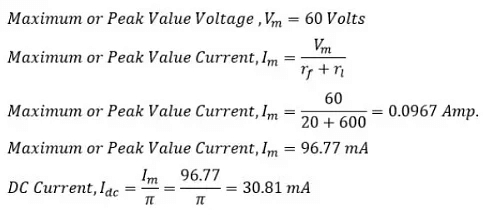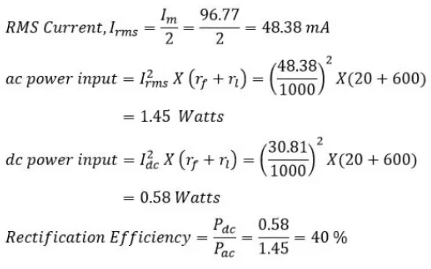Example 3: An ac supply of 230 volts is applied to step down transformer of 230/15 volts whose secondary is connected to the half wave rectifier.
Find
(1) The output DC Voltage
(2) PIV of diode.

RMS value of Tr. Secondary Voltage, Vrms = 15 Volts
Peak or Max. Secondary Voltage, Vm = Vrms X√2 = 15 X√2 = 21.21 V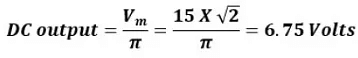Peak inverse voltage (PIV) of diode = V= 21.21  Volts

The document Single Phase Half Wave Rectifier - Notes | Study Power Electronics - Electrical Engineering (EE) is a part of the Electrical Engineering (EE) Course Power Electronics.
All you need of Electrical Engineering (EE) at this link: Electrical Engineering (EE)

## Power Electronics

5 videos|39 docs|63 tests
 Use Code STAYHOME200 and get INR 200 additional OFF

## Power Electronics

5 videos|39 docs|63 tests

Track your progress, build streaks, highlight & save important lessons and more!

,

,

,

,

,

,

,

,

,

,

,

,

,

,

,

,

,

,

,

,

,

;